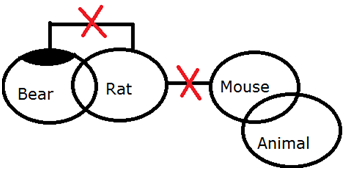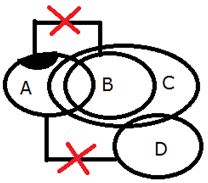# IBPS / LIC Clerk Prelims Reasoning Ability Questions 2019 (Day-02)

Dear Aspirants, Our IBPS Guide team is providing new series of Reasoning Questions for IBPS Clerk Prelims 2019 so the aspirants can practice it on a daily basis. These questions are framed by our skilled experts after understanding your needs thoroughly. Aspirants can practice these new series questions daily to familiarize with the exact exam pattern and make your preparation effective.

Check here for IBPS Clerk Prelims Mock Test 2019

##### Check here for IBPS Clerk  Mock Test 2019

[WpProQuiz 7214]

Puzzle

Directions (1-5): Study the following information carefully and answer the below questions.

There are eight boxes from A to H. They are placed on 8 different shelves but not necessarily in the same order. The lowermost shelf is numbered as 1 and the shelf above it is numbered as 2 and so on.

Box G is placed at one of the even numbered shelf, but not on shelf 6. Three boxes are placed between Box G and Box F. Box B is placed at one of the odd number shelf but not on immediately below or immediately above of both Box G and F’s shelves. More than three boxes are placed between Box D and Box E. Only one box is placed between Box A and Box H.  Box H is not placed adjacent to Box G. Number of boxes between Box C and Box F is same as Number of boxes between Box F and Box D. Box C is not placed immediately above Box A.

1) Which among the following box is placed on shelf number 5?

a) H

b) F

c) C

d) A

e) None of these.

2) How many boxes are placed between Box D and Box C?

a) 2

b) 3

c) 1

d) More than 3

e) None of these.

3) Which among the following box is placed immediately above F?

a) A

b) H

c) C

d) A

e) None of these.

4) Find the odd one out of the rest?

a) G

b) F

c) D

d) C

e) E

5) Which of the following statement is true according to E?

a) Box E is placed immediately below C.

b) Box G is placed immediately above C.

c) Box E is placed on one of the even numbered floor.

d) All are true

e) None is true

Inequality

Directions (6-8): In the following question assuming the given statements to be true, find which of the conclusion among given five conclusions.

6) Statement:

P = Q ≥ R ≤ S >  H < J ;   S < K  = L

Conclusions:

I) P ≥ K

II) H < K

a) Only Conclusion I follows

b) Only Conclusion II follows

c) Either Conclusion I or II follows

d) Neither Conclusion I nor II follows

e) Both Conclusion I and II follow

7) Statement:

A ≥ B > C = D ;  B ≤ E < F ; C > G

Conclusions:

I) A ≤ E

II) B > G

a) Only Conclusion I follows

b) Only Conclusion II follows

c) Either Conclusion I or II follows

d) Neither Conclusion I nor II follows

e) Both Conclusion I and II follow

8) Statement:

M > N < O = P ≥ S ≤ T > U = D

Conclusions:

I) N < P

II) P > D

a) Only Conclusion I follows

b) Only Conclusion II follows

c) Either Conclusion I or II follows

d) Neither Conclusion I nor II follows

e) Both Conclusion I and II follow

Syllogism

Directions (9-10): In each of the following questions, relationship between different elements is shown in the statements followed by conclusions. Find the conclusion which is definitely true.

9) Statement:

Only a few Bear are rat.

No rat is Mouse.

Some mouse are Animal.

Conclusions:

I) All Bear can never be Mouse.

II) All mouse can never be Bear

a) Only Conclusion I follows

b) Only Conclusion II follows

c) Either Conclusion I or II follows

d) Neither Conclusion I nor II follows

e) Both Conclusion I and II follow

10) Statement:

Only a few A are B.

All B are C.

Some C are D.

No A is D.

Conclusions:

I) All D can be B.

II) Some C is A.

a) Only Conclusion I follows

b) Only Conclusion II follows

c) Either Conclusion I or II follows

d) Neither Conclusion I nor II follows

e) Both Conclusion I and II follow

Directions (1-5):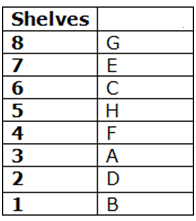Box G is placed at one of the even numbered shelf, but not on shelf 6. Three boxes are placed between Box G and Box F.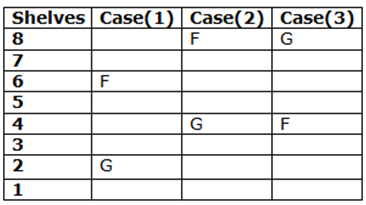Box B is placed at one of the odd number shelf but not on immediately below or immediately above of both Box G and F’s shelves.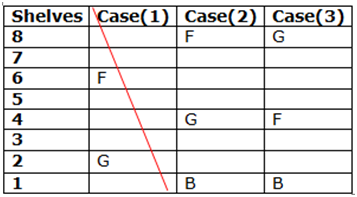Case (1) gets cancelled because Box B is not on immediately below or immediately above of both Box G and F’s shelves.

More than three boxes are placed between Box D and Box E. Only one box is placed between Box A and Box H.  Box H is not placed adjacent to Box G.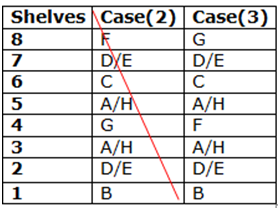Case (2) gets cancelled because H is not the immediate neighbour of G.

Number of boxes between Box C and Box F is same as Number of boxes between Box F and Box D. Box C is not placed immediately above Box A.

Hence case (3) will be the final arrangement.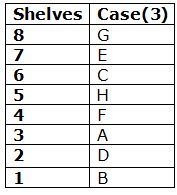Directions (6-8):

P = Q ≥ R ≤ S < K

I) P ≥ K (Conclusion I does not follows)

H < S < K

II) H < K (Conclusion II follows)

A ≥ B ≤ E

I) A ≤ E (Conclusion I does not follows)

B > C > G

II) B > G (Conclusion II follows)

N < O = P

I) N < P (Conclusion I follows)

P ≥ S ≤ T > U = D

II) P > D (Conclusion II does not follows)

Directions (9-10):